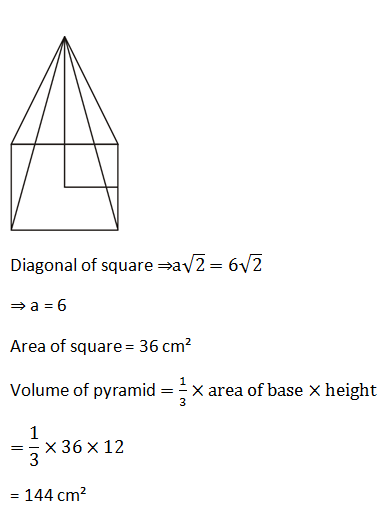# SSC Quantitative Aptitude Questions (Day-51)

Dear Aspirants, you can find the Quantitative Aptitude questions with detailed explanations for the SSC exams. Nowadays the competitive level of the exam has been increasing consistently. Due to the great demand for the government job, the level of the toughness reached greater. Candidates have to enhance the preparation process in order to drive in the right path. It doesn’t need to clear the prescribed cutoff. You must have to score good marks more than the cut off marks to get into the final provisional list. Here we have updating the Quantitative Aptitude questions with detailed explanations on a daily basis. You can practice with us and measure your level of preparation. According to that you can sculpt yourself in a proper way. SSC aspirants kindly make use of it and grab your success in your career.

Start Quiz

1) What will be the amount on Rs 25000 at the rate of 20% per annum compounded yearly for 4 years?

(a) 51840

(b) 50350

(c) 53550

(d) 48750

Directions (2-5):  The table given below shows the production (in ‘000 tonnes) of five companies from 2012 to 2016.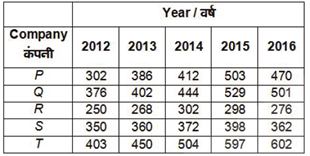2) What is the total production (in ‘000 tonnes) of company R from year 2012 to 2016?

(a) 1276

(b) 1444

(c) 1394

(d) 1502

3) What is the average (in ‘000 tonnes) of total production all companies in year 2013?

2013’000?

(a) 371.1

(b) 373.2

(c) 378.4

(d) 362.3

4) Production of company R in year 2014 is how much percent more than the production of company R in year 2013?

(a) 12.68

(b) 11.56

(c) 14.42

(d) 15.31

5) Production of all companies in year 2014 is how much percent less than the production of all companies in year 2015?

(a) 8.25

(b) 11.3

(c) 14.4

(d) 12.5

6) What is the length (in metres) of the longest rod that can be placed in a room which is 2 metres long, 2 metres broad and 6 metres high?

(a) 8

(b) 2√11

(c) 3√11

(d) 10

7) If the height of a given cone became thrice and the radius of the base remains the same. What is the ratio of the volume of the given cone and thevolume of the second cone?

(a) 1 : 3

(b) 1 : 9

(c) 1 : √3

(d) 1 : 27

8) If the angles of a triangle are (2x – 8)o, (2x + 18)o and 6xo. What is the value of 3x (in degrees)?

(a) 17

(b) 34

(c) 51

(d) 60

9) Two identical circles each of radius 2 cm intersect each other such that the circumference of each one passes through the centre of the other. What isthe area (in cm(b) of the intersecting region?

(a) 8π/3 – 2√3

(b) 8π/3 – √3

(c) 4π/3 – √3

(d) 4π/3–2√3

10) What is the volume (in cm3) of a right pyramid of height 12 cm and having a square base whose diagonal is 6√2 cm?

(a) 864

(b) 432

(c) 144

(d) 288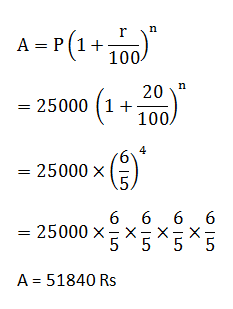Total production of company R form year

2012 to 2016 = 250 + 268 + 302 + 298 + 276 = 1394

Total production of all companies in year 2013

= 386 + 402 + 268 + 360 + 450

= 1866

Production of company R in year 2014 = 302

Production of company R in year 2013 = 268

% (more) = 34/268 × 100

= 12.68%

Production of all companies in year 2014

= 412 + 444 + 302 + 372 + 504 = 2034

Production of all companies in year 2015

= 503 + 529 + 298 + 398 + 597 = 2325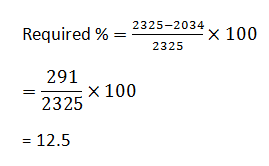Given,

ℓ = 2m,

b = 2m,

h = 6m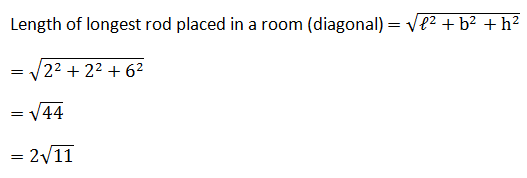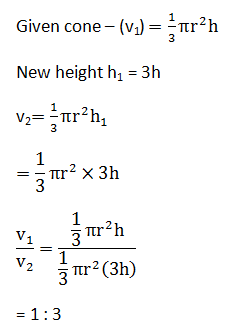We know ∠A + ∠B + ∠C = 180°

We have (2x – 8) + (2x + 18) + 6x = 180°

= 10x = 180 – 10

x = 17

3x = 3 × 17

= 51°

The area of intersecting region